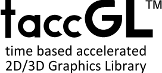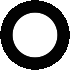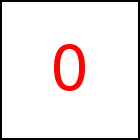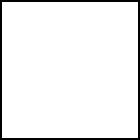# Basic Shapes - taccGL™ Tutorial

This section shows how to draw some basic shapes.

### Rectangles

The following examples draw rectangular boxes of given color, position, and size. The first example gives the position of the box using position(100,1000,0) whereby the three numbers give absolute coordinates (so you might need to scroll to find the rectangle) in pixels measured from the top left point of the document. Since this example is working in 2D, the third coordinate, the z-coordinate is 0. In the second example the position is given relatively to an HTML element with id=exd1 using {rx:0.5, ry:0.5}. The given values are multiplied by the elements width and height, so that this example positions the rectangle in the middle of the HTML element. The third example uses {ox:75, oy:60}. The values give an offset to the position of the HTML element measured in pixels.

The specification of color and size are straight forward, all numbers given to resize are measured in pixels. Finally note that we use the method a instead of actor (as it was used in the first example). a instead of actor are similar methods taking similar parameters. The difference is that actor automatically takes care about the texture, which is something we explain later on in section Integration of HTML and WebGL and which is needed when drawing HTML elemnents but not needed to draw colors.

 taccgl.a('exd1') . position(100,1000,0). color("green") . resize(200,500). duration(5). start(); RUN taccgl.a('exd1') . position({rx:0.5, ry:0.5}). color("red") . resize(20,50). duration(5). start(); RUN taccgl.a('exd1') . position({ox:75, oy:60}). color("blue") . resize(20,50). duration(5). start(); RUN

### Images, Text, 2D Drawings, Frames ... - via HTML Elements

taccgl™ can draw HTML elements. It does not have an explicit interface to draw basic shapes like Images, Text, Frames etc. Instead the idea is to define such items as HTML elements formatting them using well known HTML and CSS and then use taccgl™ functions to animate those HTML elements with the power and performance of WebGL™ possibly as integrated part of a 3D scene.The following example draws an image using taccgl™. It is defined as HTML-img tag and gets an id="exh10i". Then taccgl.actor('exh10i') selects that element, and ({rx:0.5}) moves it to the right in the middle of the original HTML element.

 taccgl.actor('exh10i') . position ({rx:0.5}). duration(5). start(); RUN

Often you will use CSS visibility:hidden (not display:none) for such HTML elements, to show them only as part of the 3D animation. Within most of the examples we don't for better illustration.

taccgl™ and WebGL™ need to allocate texture space for drawing HTML elements. Because that is limited the examples above work only if the drawn element is within the top 1424 and left 1200 pixels of the HTML document. Also drawn elements must not overlap. See Integration of HTML and WebGL and HTML Elements on Canvas for details.

### Parallelogram

To draw a parallelogram, you can specify height and width in vector form. In the first example below the height is specified as a vector hV(50,100,0) (height-vector). This specifies length and direction of the left and right edges of the parallelogram. Length and direction of the upper and lower edge are given using wV(100,0,0) (width-vector). All numbers specify length in pixels.

 taccgl.a('exd2') . color("red") .hV (50, 100, 0) .wV(100,0,0). duration(5). start(); RUN taccgl.a('exd2') . color("blue") .hV (0, 100, 0) .wV(100,100,0). duration(5). start(); RUN

### Triangle

To draw a triangle specify taccgl.triangle as second parameter of a. This will by default draw the upper left triangle of the underlying HTML element. Using hV, e.g. hV(50,100,0) (height-vector) and wV, e.g. wV(100,0,0) (width-vector) the coordinates of the 2nd and 3rd point of the triangle can be specified. All numbers specify length in pixels, relative to the first point of the triangle.

 taccgl.a('exd25',taccgl.triangle) . color("red") . duration(5). start(); RUN taccgl.a('exd25',taccgl.triangle) . color("blue") .hV (0, 100, 0) .wV(100,100,0). duration(5). start(); RUN

### Circle

The following example shows how to draw a circle in taccgl™. In fact as circle is an instance of a flexible object, so this is why the a-method has a second parameter selecting a taccgl.flexiBorder and then later on Circle () is selected. It is also possible to draw an ellipse by resizing the circle accordingly.

 taccgl.a('exd3',taccgl.flexiBorder) . Circle () . color("green"). duration(5). start(); RUN taccgl.a('exd3',taccgl.flexiBorder) . Circle () . color("green"). resize(80,170). duration(5). start(); RUN taccgl.a('exd3',taccgl.flexiBorder) . Circle () . color("green"). hV (0, 100, 0) .wV(100,100,0). duration(5). start(); RUN

Because the GPU cannot draw a circle itself (in fact the GPU just does triangles), the circle is approximated as a couple of rectangles. Using nparts you can specify how many:

 taccgl.a('exd3',taccgl.flexiBorder) . Circle () . color("green"). nparts(2). duration(5). start(); RUN taccgl.a('exd3',taccgl.flexiBorder) . Circle () . color("green"). nparts(10). duration(5). start(); RUN taccgl.a('exd3',taccgl.flexiBorder) . Circle () . color("green"). nparts(50). duration(5). start(); RUN

### Box

Using taccgl.dddBox a box can be created. Here you need to specify a color for each of the surfaces. Using selLeft, selTop ... a surface of the box can be selected and then using color a color is chosen.

Per default a box has the same width and height as the underlying HTML element. The depth defaults to the height of the HTML element. You can set width and height using resize and depth using depth.

hV and wV can be used as described above and in addition there is dV to specify the length and direction of the depth of the box in vector form.

 taccgl.a("exd4",taccgl.dddBox) .selLeft().color("green").selRight().color("brown") .selTop().color("red") .selBottom().color("yellow").selBack().color("white") .selFront().color("blue") .rotateMiddle(1,1,-1) .dur(5).start() RUN taccgl.a("exd4",taccgl.dddBox) .selLeft().color("green").selRight().color("brown") .selTop().color("red") .selBottom().color("yellow").selBack().color("white") .selFront().color("blue") .depth(10).rotateMiddle(1,1,-1) .dur(5).start() RUN taccgl.a("exd4",taccgl.dddBox) .selLeft().color("green").selRight().color("brown") .selTop().color("red") .selBottom().color("yellow").selBack().color("white") .selFront().color("blue") .hV(50,170,0) .rotateMiddle(1,1,-1) .dur(5).start() RUN taccgl.a("exd4",taccgl.dddBox) .selLeft().color("green").selRight().color("brown") .selTop().color("red") .selBottom().color("yellow").selBack().color("white") .selFront().color("blue") .dV(0,-200,-100) .rotateMiddle(1,1,-1) .dur(5).start() RUN

### Cylinder

A cylinder is created, just as a circle that was describe above, as a flexible object. It uses the flip method, that creates a kind of page flip effect by rolling up the underlying element. The following 2 examples create cylinders, the then following examples roll up partially and so create partial cylinders.

 taccgl.a("exd5",taccgl.flexiBorder) .color("#00c000"). nparts(50) .Flip(0,0,6*Math.PI/4,6*Math.PI/4) .rotateMiddle(1,0,0) .dur(5).start() RUN taccgl.a("exd5",taccgl.flexiBorder) .color("#00c000"). nparts(50) .Flip(0,0,6*Math.PI/4,6*Math.PI/4) .wV(570,0,0).hV(0,0,-170).dV(0,570,0). rotateMiddle(1,0,0) .dur(5).start() RUN taccgl.a("exd5",taccgl.flexiBorder) .color("#00c000"). nparts(50) .Flip(0,0,2*Math.PI/4,2*Math.PI/4) .rotateMiddle(1,0,0) .dur(5).start() RUN taccgl.a("exd5",taccgl.flexiBorder) .color("#00c000"). nparts(50) .Flip(0,0,1*Math.PI/4,2*Math.PI/4) .rotateMiddle(1,0,0) .dur(5).start() RUN taccgl.a("exd5",taccgl.flexiBorder) .color("#00c000"). nparts(50) .Flip(.5,0,0,0) .rotateMiddle(1,0,0) .dur(5).start() RUN

WebGL™ is a trademark of the Khronos Group Inc.

Next Page:Basic 3D Models
Previous Page: First Example

# Tutorial

taccgl™ - Canvas Library